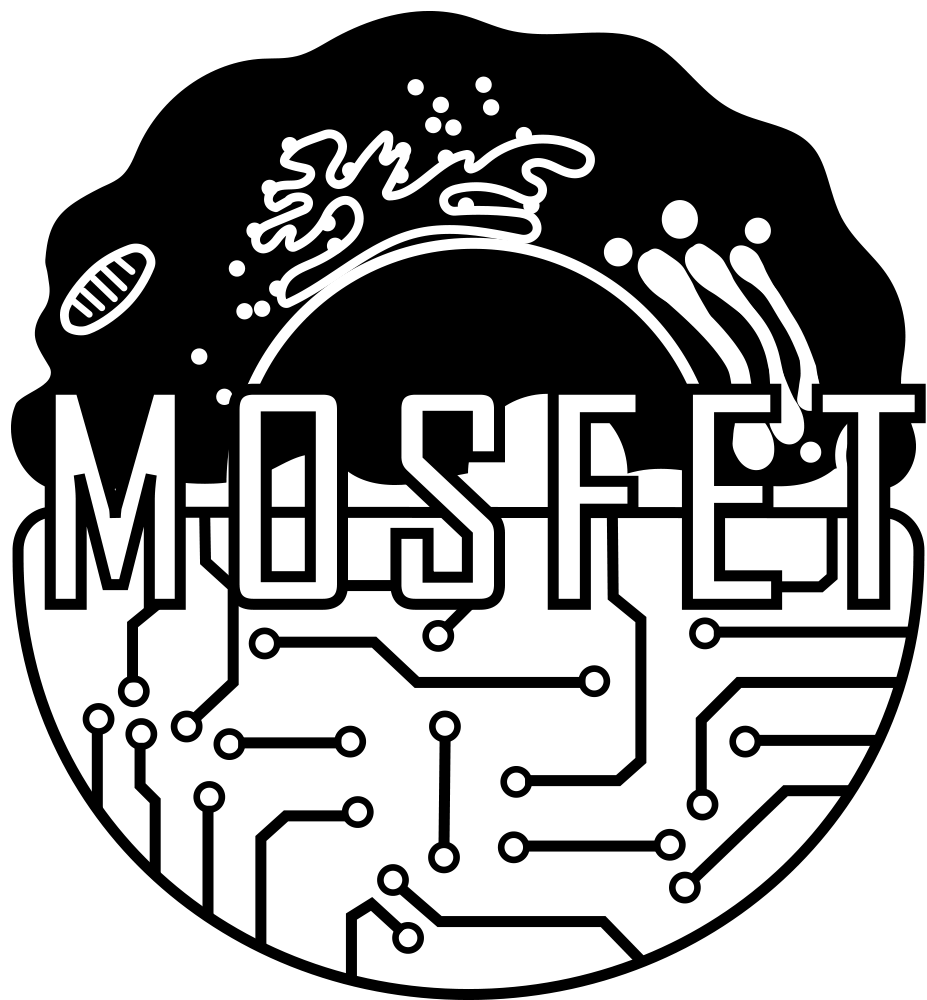# Team:NAU-CHINA/Auxiliary Understanding

Template:2018_NAU-CHINASafety

Model

Auxiliary Understanding# Part1 Why we use stochastic simulation

Ordinary differential equation is a commonly used mathematical tool to describe the chemical reaction. It can be accurate to use the description of the ordinary differential equations, if the simulated system contains more than 10 ^ 3 molecules. However, it is sometimes inappropriate when applying in biological systems, because the life behavior of biochemical reactions involved in small number of molecules. For example, in the gene expression, there is usually only one kind of protein, while a few dozen mRNA molecules, corresponding to these genes. In protein interactions, the number of proteins as reactant is small, far less than the number of molecules in ordinary chemical reactions. The time of chemical reaction in organisms, on the other hand, is longer than that of ordinary chemical reactions. For example, it usually takes a few minutes to complete gene transcription process. The small number of molecules and their slow reaction produces large randomness in chemical reactions. This randomness is caused by two factors. Firstly, the reactant collision response may occur. When the molecules are in a small number or in low concentration, the reactant collision probability is very small. Another factor is a thermodynamic fluctuation. Even in reaction of reactant collision together, the activation energy, which is affected by the fluctuation of heat, has a significant randomness.

# Part2 Gillespie algorithm（stochastic simulation algorithm）

We are the users of the algorithm, not the inventor, and the following literature is the explanation made by the algorithm inventor.

"It is a relatively simple digital computer algorithm which uses a rigorously derived Monte Carlo procedure to numerically simulate the time evolution of the given chemical system."

Exact stochastic simulation of coupled chemical reactions

Daniel T. Gillespie

The Journal of Physical Chemistry 1977 81 (25), 2340-2361

DOI: 10.1021/j100540a008